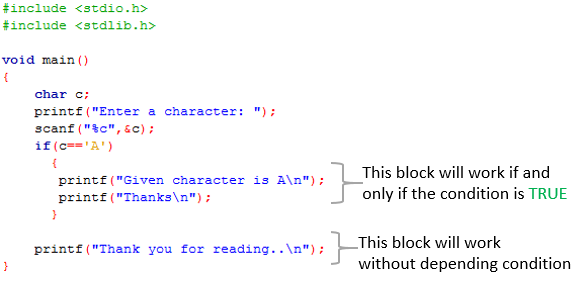## C

Simplified tutorials to learn the popular basic language C

## VB.Net

Learn the basic desktop development application language

## CodeIgniter

One of the popular PHP three tier (MVC) framework

# If else Statement in C Programming

Abstraction:
This tutorial describes the concept of 'else if statement' in C programming with examples

You are already familiar with one of the IF statementsSimple IF. You know if statements are using for making decisions though conditions.

There are 4 types of IF statements
• Simple if statement
• If - else statement
• Nested if - else statement
• Else - if ladder
Simple if statement:

We already learnt about simple if statement in previous lesson

if – else statement

In simple if statement, there is no statement block to work when the if condition is false. That is, that does not describe anything when the condition is false. Where as in if – else statement, here is an else part, it describes what to work if the if condition is false

Let us learn with a normal English statement:

 If he pass in final exam, then he is a winner else he is a loser

Here he will be a winner, if and only if he pass in final exam, else (if he fail in exam) he is a loser.

In method of C language:
 If ( he pass in final exam)  {     He is a winner  }  else  {     He is a loser.   }

Another example :
• To check a whether a number is positive or not.
Here logic to check whether a number is positive is, if the given number is greater than 0,then the given number is positive else not.

In English language:
 If the given number is greater than zero Then the given number is positive Else the given number is NOT a positive

Come to method of C language:

 If(given number is greater than zero)   {        The given number is positive   }   Else   {        The given number is not a positive   }

Implementing above problem as a C program: (With Code blocks)

 #include #include void main()  {   int number;   printf("Enter a number: ");   scanf("%d",&number);   if(number>0)    {      printf("Given number is positive\n");    }   else    {      printf("Given number is NOT positive\n");     }   }

Code Explanation:

Here if the condition is true: the given number is greater than zero (number>0), then the statement [printf("Given number is positive\n");] will work. If the condition is false, then the else part will work. [ printf("Given number is NOT positive\n");]

Output when the Condition is True

Output when the Condition is False

NOTE:

Here both if part and else part contain only one sentence, so no need the brace symbol. So it is good to remove useless braces.

So above program is equivalent to this (no braces):

 #include #include void main()  {   int number;   printf("Enter a number: ");   scanf("%d",&number);   if(number>0)        printf("Given number is positive\n");   else       printf("Given number is NOT positive\n"); }

If if or else block contain more than one statement, then you should use the brace, or the only the first statement will work.

Let us see with another example:
• Program to check whether the given number is 100 or not. if it is 100, then display the given number and its double.
 #include #include void main()  {   int number;   printf("Enter a number: ");   scanf("%d",&number);    if(number ==100)    {       printf("Given number is 100\n");       printf("Its double is %d\n\n",2*number);      }    else      printf("Given number is NOT 100\n"); }

Here if part contains two statements, so we use brace and else block contains only one statement, so no need the brace symbol.

Difference between simple if and if – else statement:
• In simple if statement, that contains a block of statements to work if and only if the condition is true. There is no statement if the condition is false (there is no else part).
• Where as in if-else statement, there are statement block to work if the condition is true and false.
• If we write some statements out of the condition, then they will work when both the condition is true or false. That is, they do not depend the condition.
Differentiate the output of both below programs:

Simple if:Output when condition is FALSE

if-else:

Output when condition is TRUE

Output when condition is FALSE